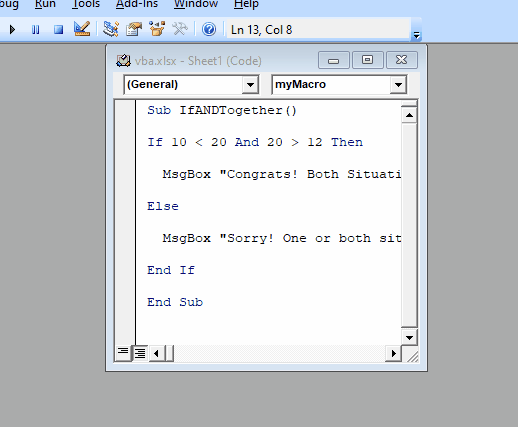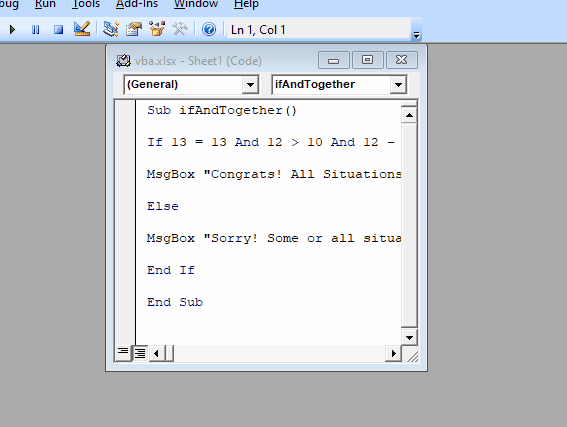# VBA Use AND Operator in IF Statement

We will introduce how to use the `IF` statement and the Operator `AND` together in VBA with examples.

## Use `AND` Operator on `If` Statement in VBA

The function of the `AND` operator will return `True` only when all the operator conditions are `True`. If any of the conditions from the `AND` operator is `False`, it will return `False`.

We can use the `AND` operator with an `If` statement, which gives one value if the condition is `True` and gives another value if the condition is `False`.

VBA allows combining the `AND` function with VBA `If` statement. Multiple conditions can be tested by combining two functions in VBA coding.

The outcome comes to be `True` if both functions are true simultaneously and turns out as `False` if the outcome of any of the two functions is false at a point. We can combine the If and AND functions by following the following steps below.

1. Code starts with the `If` keyword.
2. The first condition is specified for the `If` function.
3. `AND` keyword is used to specify the second function.
4. We will define the second condition for the `AND` function.

Let’s have an example in which we will use both `If` and `AND` together.

Code:

``````# vba
Sub IfANDTogether()

If 10 < 20 And 20 > 12 Then

MsgBox "Congrats! Both Situations are passed"

Else

MsgBox "Sorry! One or both situations have failed."

End If

End Sub
``````

Output:As you can see, when both conditions are `True`, we receive a congratulations message. But when one or both situations are `False`, we receive a message of sorry.

We can also use more than two conditions, as shown below.

Code:

``````# vba
Sub ifAndTogether()

If 13 = 13 And 12 > 10 And 12 - 1 = 11 Then

MsgBox "Congrats! All Situations are passed."

Else

MsgBox "Sorry! Some or all situations have failed."

End If

End Sub
``````

Output:The `AND` operator can help us with multiple conditions to get the desired result.

Write for us
DelftStack articles are written by software geeks like you. If you also would like to contribute to DelftStack by writing paid articles, you can check the write for us page.